# Quick Tip: Collision Detection Between a Circle and a LineThis post is part of a series called Collision Detection and Reaction.
Quick Tip: Collision Detection Between Circles
Quick Tip: Collision Detection Between a Circle and a Line Segment

In my previous Quick Tip, we looked at the idea of collision detection in general, and specifically at detecting collisions between a pair of circles. In this Quick Tip, we'll look at detecting a collision between a circle and a line.

This is the result that we will work on. Click the Restart button to reposition all circles at the top of the stage and watch them fall down.

Note that the circles collide with the line even outside of the segment that is drawn. My next Quick Tip will show how to fix this.

## Step 1: The General Idea

To check whether any circle has collided with a line, we have to check the perpendicular length from the line to circle. Observe the diagram below.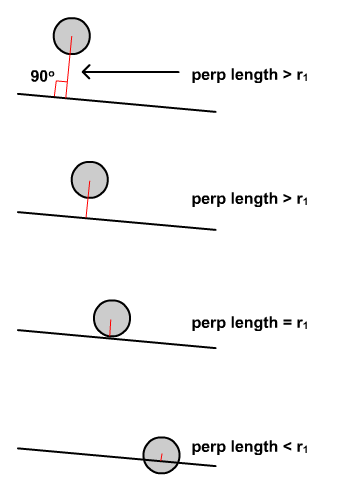It is clear from the diagram above that cases 3 and 4 should detect a collision between the circle and the line. So we conclude that if the perpendicular length (marked in red) is equal or less than circle's radius, a collision happened due to the circle touching or overlapping the line. Question is, how do we calculate this perpendicular length? Well, Vectors can help to simplify our problem.

## Step 2: Line Normal

In order to draw a line on stage, we need two coordinates (c1 and c2). The line drawn from c1 to c2 will form a vector pointing to c2 (Note the direction of the arrow).

Next, we need to find the line's normal. The line's normal is another line that makes 90° with the original line, and intersects with it at a point. Despite the line's normal being yet another line, the normal's vector form can be further identified as the left or right normal relative to the line's vector. The left normal is the line vector itself, rotated -90°. The right normal is the same but rotated 90°. Remember, the y-axis in Flash's coordinate space is inverted compared to the y-axis on a typical graph - so positive rotation is clockwise and negative rotation is counter-clockwise.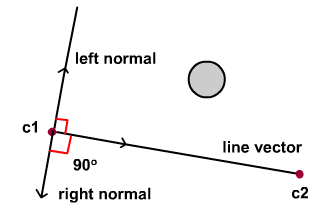## Step 3: Projection on Left Normal

The left normal is used in our attempt to calculate the perpendicular length between the circle and the line. Details can be found in the diagram below. A refers to a vector pointing from c1 to the circle. The perpendicular length actually refers to vector A's projection on the left normal. We derive this projection by using trigonometry: it is |A| Cosine (theta), where |A| refers to the magnitude of the vector A.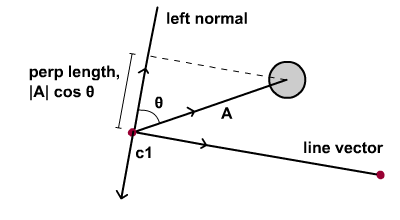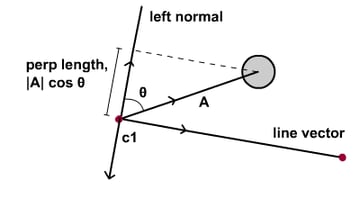The simplest approach is to make use of vector operations, specifically the dot product. Starting from the equation of the dot product, we rearrange the terms so that we arrive at the second expression shown below. Note that the right side of the second equation is the projection that we wanted to calculate!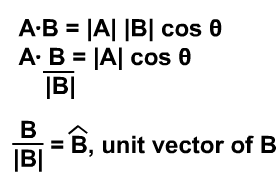Also note the left and right side of the equation will ultimately produce the same result, although different in their approaches. So instead of using the right side of the equation, we can opt for the left side of equation. To easily arrive at the end result, it is favourable to use the left because variables can be easily resolved. If we insist on using the right of equation, we'd have to push ActionScript through rigourous Mathematical work in calculating the angle theta. We conclude with the diagram below.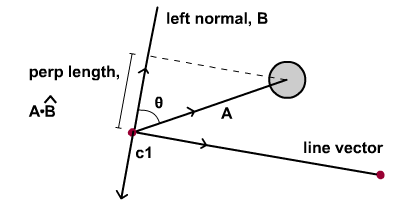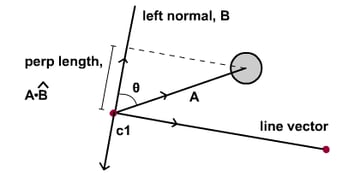(*Additional note: If the circle falls below line vector, the perpendicular length calculated from the formula in above diagram will produce a negative value.)

## Step 4: Implementing Circle-Line Collision Detection

Now that we have understood the approach mathematically, let's proceed to implement it in ActionScript. In this first section, note the line's vector is being rotated -90° to form the left normal.

In this second section, I have highlighted the calculations mentioned and the condition to check for collision between circle and line.

For those who'd like to investigate further, the following are excerpts of methods used in Vector.as

## Step 5: The Result

Press the Restart button to reposition all circles at the top of stage. Note that the collision is between the whole line (including the section not drawn) and the circles. In order to limit collision to line segment only, stay tuned for the next Quick Tip.

## Conclusion

Thanks for reading. Stay tuned for the next tip.